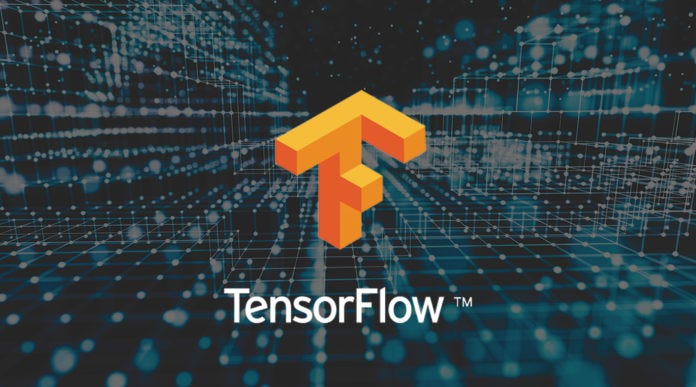# Getting Started with TensorFlowTensorFlow makes it easy for beginners and experts to create machine learning models for desktop, mobile, web, and cloud. See the sections below to get started. Python programs are run directly in the browser—a great way to learn and use TensorFlow.

The mathematical concept of a tensor could be broadly explained in the following way: If a scalar has the lowest dimensionality and is followed by a vector and then by a matrix. A tensor would be the next object in the line. Scalar, vectors and matrices are all tensors of rank 0, 1 and 2 respectively. Tensors are simply a generalization of the concepts we have seen so far.

## How TensorFlow Works

At first, computation in TensorFlow may seem needlessly complicated. But there is a reason for it: because of how TensorFlow treats computation, developing more complicated algorithms is relatively easy. We will look into the pseudocode of a TensorFlow algorithm.

### How to do it…

we will introduce the general flow of TensorFlow algorithms.

1. Import or generate datasets: All of our machine-learning algorithms will depend on datasets. We will either generate data or use an outside source of datasets. Sometimes it is better to rely on generated data because we will just want to know the expected outcome.
2. Transform and normalize data: Normally, input datasets do not come in the shape TensorFlow would expect so we need to transform TensorFlow them to the accepted shape. We will have to transform our data before we can use it. Most algorithms also expect normalized data and we will do this here as well. TensorFlow has built-in functions that can normalize the data for you as follows:
3. data = tf.nn.batch_norm_with_global_normalization(…)
4. *Partition datasets into train, test, and validation set: *We generally want to test our algorithms on different sets that we have trained on.
5. Set algorithm parameters (hyperparameters): Our algorithms usually have a set of parameters that we hold constant throughout the procedure. For example, this can be the number of iterations, the learning rate. It is considered good form to initialize these together so the reader or user can easily find them, as follows:
6. learning_rate = 0.01
7. batch_size = 100
8. iterations = 1000
9. Initialize variables and placeholders: TensorFlow depends on knowing what it can and cannot modify. TensorFlow will modify/adjust the variables and weight/bias during optimization to minimize a loss function. To accomplish this, we feed in data through placeholders. We need to initialize both of these variables and placeholders with size and type so that TensorFlow knows what to expect. TensorFlow also needs to know the type of data to expect.

avar = tf.constant(42)_

xinput = tf.placeholder(tf.float32, [None, input_size])_

yinput = tf.placeholder(tf.float32, [None, num_classes])_

6. *Define the model structure: *After we have the data, and have initialized our variables and placeholders, we have to define the model. This is done by building a computational graph. TensorFlow chooses what operations and values must be the variables and placeholders to arrive at our model outcomes.

7. Declare the loss functions: After defining the model, we must be able to evaluate the output. This is where we declare the loss function. The loss function is very important as it tells us how far off our predictions are from the actual values.

loss = tf.reduce_mean(tf.square(y_actual — y_pred))

8.*Initialize and train the model: *Now that we have everything in place, we need to create an instance of our graph, feed in the data through the placeholders, and let TensorFlow change the variables to better predict our training data.

with tf.Session(graph=graph) as session:

session.run(…)

Note that we can also initiate our graph with:

session = tf.Session(graph=graph)

session.run(…)

## Deep Learning 101 —  Neural Networks Explained

The past few decades have witnessed a massive boom in the penetration as well as the power of computation, and amidst this information.

## Artificial Neural Networks — Recurrent Neural Networks

Artificial Neural Networks — Recurrent Neural Networks. Remembering the history and predicting the future with neural networks. A intuition behind Recurrent neural networks.

## Deep Learning with scikit-learn

Deep Learning with scikit-learn: PyTorch, TensorFlow and Caffe aren’t the only frameworks for Deep Learning. There is also a l library with a scikit-learn like API.

## Learn TensorFlow.js - Deep Learning and Neural Networks with JavaScript

This full course introduces the concept of client-side artificial neural networks. We will learn how to deploy and run models along with full deep learning applications in the browser! To implement this cool capability, we’ll be using TensorFlow.js (TFJS), TensorFlow’s JavaScript library.

## Deep Neural Network Language Identification

Classify the language of a piece of text using a DNN and character tri-grams. Language identification can be an important step in a Natural Language Processing (NLP) problem.